Practice Papers of Mathemetical Skills and logical Reasoning

Name
1
Find the next figures in the pattern given below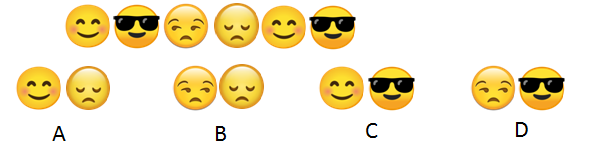Deselect Answer
2
The weight of pomegranates is ____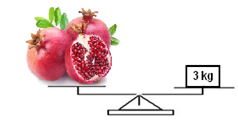Deselect Answer
3
If Rahul’s summer holidays started from 2ndThursday of June, then on which date did Rahul’s summer holidays start?Deselect Answer
4
Find the odd one out: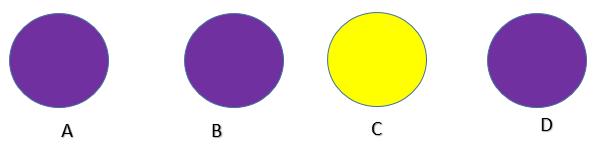Deselect Answer
5
“ZYX” as coded as “ABC” then “TUV” is coded as _____
6
Which one of the following is the smallest tree?Deselect Answer
7
_________ will replace the (?) in the given image pattern.Deselect Answer
8
Find the missing number, if same rule is followed in the given figure.Deselect Answer
9
Is ‘Sunday’ is called ‘Monday’, ‘Monday’ is called ‘Tuesday’, ’Tuesday’ is called ‘Wednesday’. Then which of these comes just after Monday?
10
__________ is the fattest boy and _______ is the thinnest boy.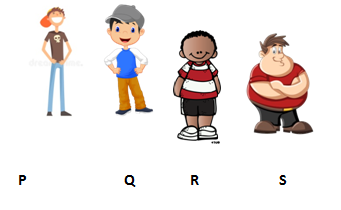Deselect Answer
11
Which of the following option does not follow the same pattern followed in the given image?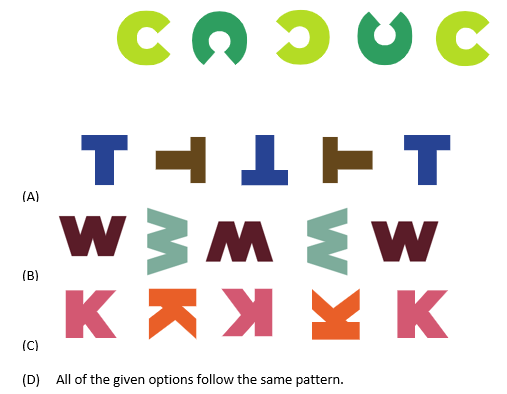Deselect Answer
12
Which of the following eggs is the heaviest?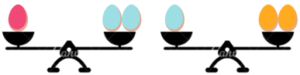Deselect Answer
13
How many groups of 5 apples (if there are 3 apples in each plate) can be formed from these apples?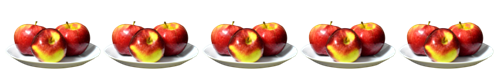Deselect Answer
14
Identify the rule followed in the number series given below.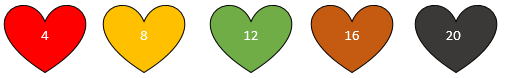Deselect Answer
15
The beaker has _____ ml of water in it.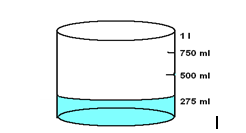Deselect Answer
16
In a certain code language, the letters are coded as shown here.Deselect Answer
17
If ‘foot’ is called ‘hand’, ‘hand’ is called ‘nose’, ‘nose’ is called ‘forehead’, then from which organ you breathe?
18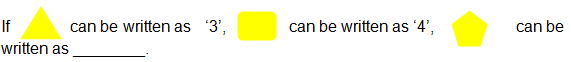Deselect Answer
19
If ‘green’ is called ‘blue’, ‘blue’ is called ‘orange’, ‘orange’ is called ‘red’, then what is the color of sky?
20
There is a certain relation between two shapes given on one side of :: . Out of the below four alternatives, one Shape will have similar relation with the Shape on other side of :: .Deselect Answer